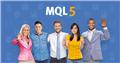# Calculation of Barsala's bankruptcy probability25

In the EA of MT4, from the "win rate" and "profit factor" of the trade result specified by the variable,I want to calculate the bankruptcy probability result based on Barsala's bankruptcy probability table.

I would be grateful if you could provide a code to calculate the EA based on Balsala's bankruptcy probability table, or a code that reads and calculates a file from an Excel file containing the Balsala's bankruptcy probability table.MQL5フォーラム
• www.mql5.com
MQL5: 自動トレーディングシステムとストラテジーテスティングに関するフォーラム